Latest Banking jobs   »   Quantitative Aptitude Quiz For SBI Clerk/IBPS...

# Quantitative Aptitude Quiz For SBI Clerk/IBPS RRB Clerk Mains 2021- 30th September

Q1. Anshul deposited two parts of a sum of Rs. 25000 in different banks at the rates of 15% per annum and 18% per annum respectively. In one year he got Rs. 4050 as the total interest. What was the amount deposited at the rate of 18% per annum?
(a) Rs. 9000
(b) Rs. 18000
(c) Rs. 15000
(d) Rs. 10000
(e) Rs. 12000

Q2. A watch dealer usually sells watches for Rs.2350 per watch. Once he gave two successive discounts of 15% and 25% while selling a watch to a customer. But he charged an additional 8% on the net sale price from the customer. By what percent is the new selling price less than the original selling price?
(a) 28.45%
(b) 29.25%
(c) 30.45%
(d) 31.15%
(e) 24.25%

Q3. P and Q started a business by investing Rs. 45,000 and Rs.54,000 respectively. After four months R joined the business with a capital of Rs. 30,000. After two more months Q left the business with his capital. At the end of the year P got a share of Rs. 13,500 in the profit. What is the total profit earned?
(a) Rs 26800
(b) Rs 27600
(c) Rs 28600
(d) Rs 29200
(e) Rs 32300

Q4. Two pipes A and B can fill a cistern in 15 hours and 10 hours respectively. A tap can empty the full cistern in 30 hours. All the three taps were open for 2 hours, when it was remembered that the emptying tap had been left open. It was then closed. How many hours more would it take for the cistern to be filled ?
(a) 4hr 30 min.
(b) 4hr 12 min.
(c) 4 hr24 min.
(d) 4hr 35 min.
(e) 4hr 54 min.

Q5. There are five boxes. The weight of the first box is 200 kg and the weight of the second box is 20% higher than the weight of the third box, whose weight is 25% higher than the first box’s weight. The fourth box at 350 kg is 30% lighter than the fifth box. Find the difference in the average weight of the four heaviest boxes and the four lightest boxes.
(a) 72.5 kg
(b) 75 kg
(c) 36.4 kg
(d) 32 kg
(e) 67.5 Kg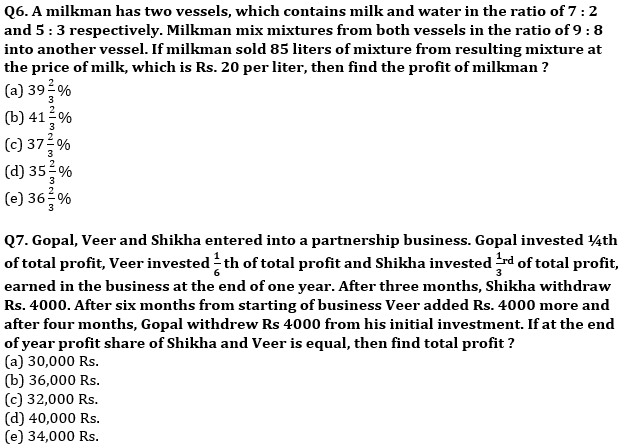Q8. A man invested an amount in the ratio of 3 : 6 : 5 at the rate of 10%, 15% and 20% respectively in three different schemes. If man gets total compound interest of Rs 9530 from all three schemes in two years then find amount invested by man at the rate of 15% ?
(a) 20000 Rs.
(b) 16000 Rs.
(c) 8000 Rs.
(d) 10000 Rs.
(e) 12000 Rs.

Q9. In 2016 a store sold some mobile phone at certain price. In 2017 store increase selling of mobile phone by reducing the price of mobile phone by 25%. The total revenue generated by selling mobile phone in 2017 is increased by 5% compared to previous year. The total revenue generated by store in 2017 is 94.5 lakh and number of mobile phones sold by store in the year 2017 is 90 more than that in 2016. Find initial cost of one mobile.
(a) 32000 Rs.
(b) 40000 Rs.
(c) 36000 Rs.
(d) 24000 Rs.
(e) 20000 Rs.

Q10. Height of a cylindrical vessel is equal to side of a square, whose area is 256 cm². Radius of cylindrical vessel is equal to average of radius of a sphere and hemisphere. If ratio between the radius of hemisphere and that of the sphere is 2 : 1 and difference between their total surface area is 4928 cm², then find volume of cylindrical vessel ?
(a) 21,288 cm³
(b) 20,176 cm³
(c) 22,176 cm³
(d) 26,176 cm³
(e) 24,288 cm³

Solutions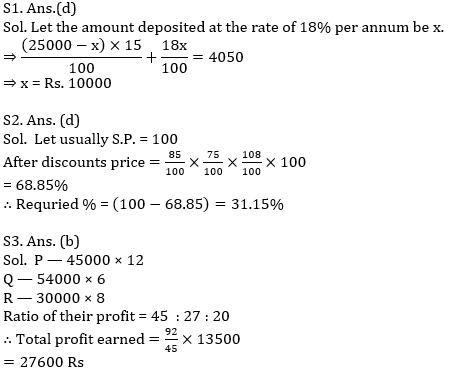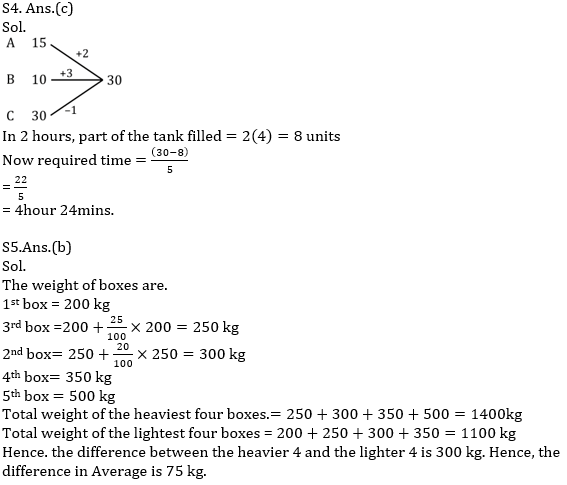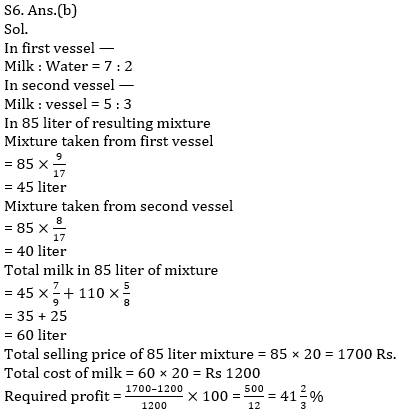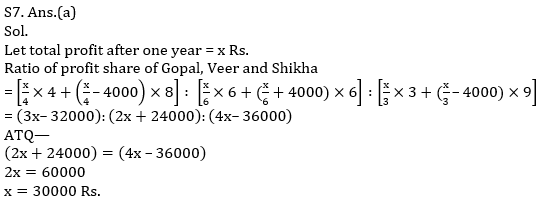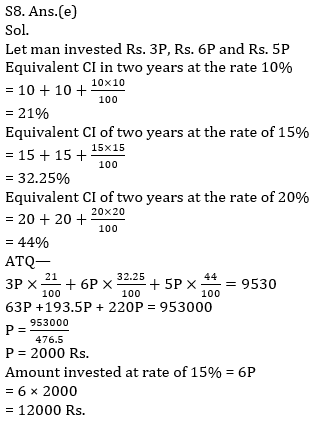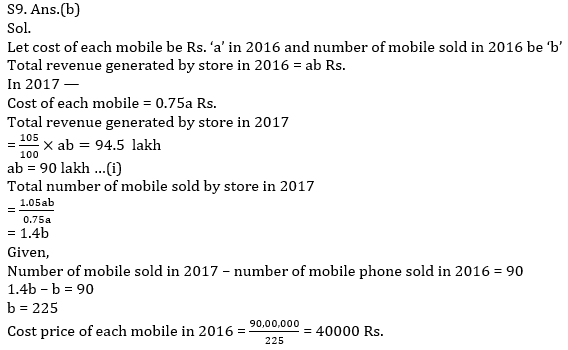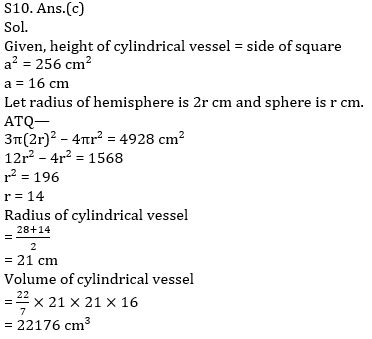Click Here to Register for Bank Exams 2021 Preparation Material#### Congratulations!Download Hindu Review of October 2021: Free PDF